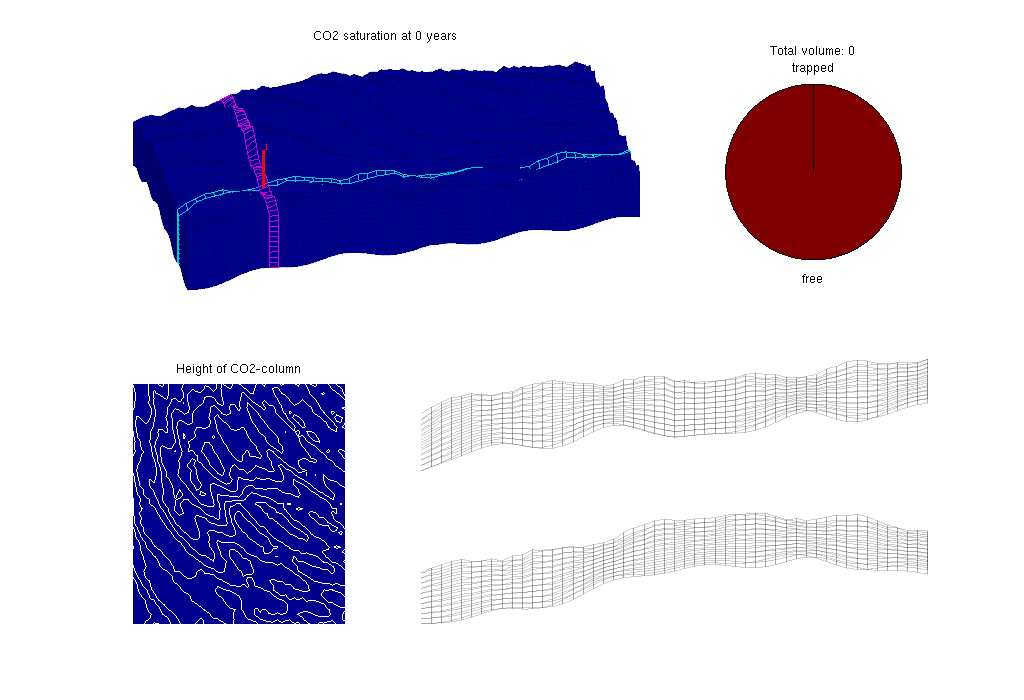#VE Simulation: Sloping Aquifer

In this example we consider a synthetic sloping aquifer, created in this tutorial. The topography of the top surface and the geological layers in the model is generated by combining the membrane function (MATLAB logo) and a sinusoidal surface with random perturbations.

Here, CO2 is injected in the aquifer for a period of 30 years. Thereafter we simulate the migration of the CO2 in a post-injection period of 720 years.

The simulation is done using the vertical average/equilibrium framework.

## Construct stratigraphic and petrophysical model

Description of how the model is created is provided in a separate tutorial.

## Set time and fluid parameters

```T          = 750*year();
stopInject = 30*year();
dT         = 1*year();
dTplot     = 5*dT;

% Make fluid structures, using data that are resonable at p = 300 bar
fluidVE = initVEFluid(Gt, 'mu' , [0.056641 0.30860] .* centi*poise, ...
'rho', [686.54 975.86] .* kilogram/meter^3, ...
'sr', 0.2, 'sw', 0.1, 'kwm', [0.2142 0.85]);
gravity on
```

## Set well and boundary conditions

We use one well placed down the flank of the model, perforated in the bottom layer. Injection rate is 1.4e3 m^3/day of supercritical CO2. Hydrostatic boundary conditions are specified on all outer boundaries.

```% Set well in 3D model
wellIx = [G.cartDims(1:2)/5, G.cartDims([3 3])];
rate   = 2.8e3*meter^3/day;
W      = verticalWell([], G, rock, wellIx(1), wellIx(2), ...
wellIx(3):wellIx(4), 'Type', 'rate', 'Val', rate, ...
'Radius', 0.1, 'comp_i', [1,0], 'name', 'I');

% Well and BC in 2D model
wellIxVE = find(Gt.columns.cells == W(1).cells(1));
wellIxVE = find(wellIxVE - Gt.cells.columnPos >= 0, 1, 'last' );
WVE      = addWell([], Gt, rock2D, wellIxVE, ...
bcVE     = addBC([], bcIxVE, 'pressure', ...
Gt.faces.z(bcIxVE)*fluidVE.rho(2)*norm(gravity));
bcVE     = rmfield(bcVE,'sat');
bcVE.h   = zeros(size(bcVE.face));

% for 2D/vertical average, we need to change defintion of the wells
for i=1:numel(WVE)
WVE(i).compi = NaN;
WVE(i).h = Gt.cells.H(WVE(i).cells);
end
```

## Prepare simulations

Compute inner products and instantiate solution structure

```SVE = computeMimeticIPVE(Gt, rock2D, 'Innerproduct','ip_simple');
preComp = initTransportVE(Gt, rock2D);
sol = initResSol(Gt, 0);
sol.wellSol = initWellSol(W, 300*barsa());
sol.h = zeros(Gt.cells.num, 1);
sol.max_h = sol.h;
```

## Prepare plotting

We will make a composite plot that consists of several parts:

• a 3D plot of the plume
• a pie chart of trapped versus free volume
• a plane view of the plume from above
• two cross-sections in the x/y directions through the well
Details of the plotting is provided in the full tutorial, ` runSlopingAquifer.m`, accompanying the VE module.## Main loop

Run the simulation using a sequential splitting with pressure and transport computed in separate steps. The transport solver is formulated with the height of the CO2 plume as the primary unknown and the relative height (or saturation) must therefore be reconstructed.

```t = 0;
while t% Advance solution: compute pressure and then transport
sol = solveIncompFlowVE(  sol, Gt, SVE, rock, fluidVE, ...
'bc', bcVE, 'wells', WVE);
sol = explicitTransportVE(sol, Gt, dT, rock, fluidVE, ...
'bc', bcVE, 'wells', WVE, 'preComp', preComp);

% Reconstruct 'saturation' defined as s=h/H, where h is the height of
% the CO2 plume and H is the total height of the formation
sol.s = height2Sat(sol, Gt, fluidVE);
assert( max(sol.s(:,1))<1+eps && min(sol.s(:,1))>-eps );
t = t + dT;

% Check if we are to stop injecting
if t>= stopInject
WVE  = []; bcVE = [];
end

% Compute trapped and free volumes of CO2
freeVol = ...
sum(sol.h.*rock2D.poro.*Gt.cells.volumes)*(1-fluidVE.sw);
trappedVol = ...
sum((sol.max_h-sol.h).*rock2D.poro.*Gt.cells.volumes)*fluidVE.sr;
totVol = trappedVol + freeVol;

% Plotting (...) details are provided in the full tutorial runSlopingAquifer.m accompanying the VE module.
end
```

Published April 20, 2009